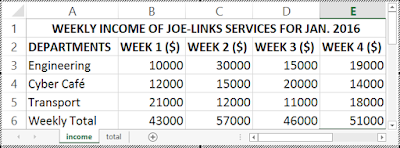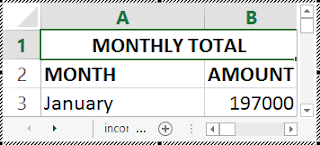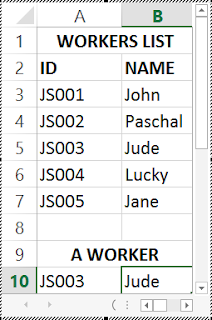You can also reference cells and formulas on other worksheets of the same workbook in Excel. In the chapter one of this tutorial, I illustrated how you can reference data, cell and formulas in the same worksheet of Microsoft Excel.
This is useful when you have related data in different worksheets of a workbook which you want to analyze in another worksheet of that same workbook.

To reference a cell in another worksheet, you begin by typing the equality sign (=), followed by the referenced worksheet name, followed by an exclamation mark also known as bang (!) and lastly, you type the absolute cell reference of the cell, e.g. A1.

The syntax for referencing a data cell in another worksheet is: =Referenced_worksheet_name!absolute_cell_reference_of_the_cell

## Practical Illustration of Referencing a Data Cell in Another Worksheet

### QUESTION:

Joe-Links Services made use of two worksheets of a workbook to record the weekly income from its various departments for the month of January 2016 as shown in the figure below. The first worksheet contains the various departments, the weekly income made by each department and the weekly total income of all the departments. The second worksheet will contain the sum of the various weekly totals. Assuming the worksheet names are Income and Total respectively, calculate the total of the weekly income inside the second worksheet (Total).Worksheet 1 - Income

### SOLUTION TO THE ABOVE QUESTION

1. Prepare the two worksheets as shown in the figures above and rename them to income and total respectively.

2. Activate the second worksheet (total) and type in any of the following formulas in cell B2:
=SUM(income!B6:income!E6) and press Enter.
OR type this:
=income!B6+income!C6+income!D6+income!E6 and press Enter.

The two functions returns the same value. The first one is a built-in function and I made use of range. While the second function is the normal addition.

income! refers to the reference worksheet name from which you get your values.
The second worksheet should now contain the returned value as shown in the figure below.## THE LOOKUP BUILT-IN FUNCTION IN EXCEL

This is an Excel built-in function that returns a data in a cell of a particular row using a provided data in another cell of that same row as reference or criteria.

For example, if you have a worksheet made up of two columns namely: ID and PRODUCT, we can use a particular ID under the ID column (say 101) to find the product name corresponding to that ID.
There are two types of LOOKUP functions in Excel namely: VECTOR LOOKUP function and ARRAY LOOKUP function, but the latter is beyond the scope of this tutorial.

The syntax for the VECTOR LOOKUP function is:
=LOOKUP(lookup_value,lookup_vector,[result_vector])

The function takes 3 arguments:

1. lookup_value (required): This is the provided data or cell reference of the provided data that Excel uses to lookup a data in another cell of the same row. Lookup_value can be a number, text, a logical value, or a name or reference that refers to a value. In the case of the example above, it will be the ID 101 or its relative cell reference e.g. A2.

2. lookup_vector (required): This is the specified range of data used to search for a particular data in that range of data. The values in lookup_vector can be text, numbers, or logical values. In the case of the above example, it will be the ID column data range e.g. A2:A10.

Note that the values in lookup_vector must be placed in ascending order: ..., -2, -1, 0, 1, 2, ..., A-Z, FALSE, TRUE; otherwise, LOOKUP might not return the correct value. Uppercase and lowercase text are equivalent.

3. result_vector (optional): This is the range of columns that contains the data that Excel returns. The result_vector argument must be the same size as lookup_vector. In the case of the above example, it will be the PROCDUCT column data range e.g. B2:B10.

#### NOTE:

When dealing with number columns, you must first sort the whole data either in ascending or descending order before typing the LOOKUP function else Excel returns an error message #N/A.
If the LOOKUP function can't find the lookup_value, the function matches the largest value in lookup_vector that is less than or equal to lookup_value.
If lookup_value is smaller than the smallest value in lookup_vector, LOOKUP returns the #N/A error value.
Now let me use a more practical illustration to get put you through.

## PRACTICAL ILLUSTRATION OF LOOKUP FUNCTION (VECTOR LOOKUP)

### QUESTION:

The figure below shows a spreadsheet that contains a list of workers and their ID. Use the LOOKUP function to find the worker with ID equal to JS003.### SOLUTION TO THE LOOKUP QUESTION

1. Prepare a worksheet as shown in the figure above.

2. Highlight the whole data (i.e. A3 to B7).

3. The ID column is already in descending order, therefore there is no need to resort it again.

4. Place your cell pointer in cell B10 and type the following formula:
=LOOKUP(A5,A3:A7,B3:B7) and press Enter.

Excel returns the name JUDE, the worker with the ID JS003 as shown in the figure below.There is more this function can do than this. I just used this illustration to put you through. Now it is time for you to utilize this knowledge in more practical problems.
Now, I will show you a similar function to LOOKUP called VLOOKUP function and how it works.
Go to the last part (Part 6) of this tutorial chapter: USING THE VLOOKUP FUNCTION TO DESIGN WORKERS PAYROLL SYSTEM IN EXCEL

Comment below if you are hooked up along the installation process.

## Recommended Entrepreneurship BooksThe Passionate Entrepreneur’s Strategies: Learn how to discover business ideas that will be successful, grow multiple online income streams, make money from home, attract new and retain customers, applications of SWOT analysis in any business, skills that will help you succeed as an entrepreneur, self-discipline, etc. Lucrative Business Ideas Series Book 1. By Buzzer Joseph. Read more about the book.How to Make Money from Blogging and Affiliate Marketing: Learn the Step by Step Guide of Creating, Growing and Optimizing your WordPress Blog from Scratch for Search Engines (SEO) and How to Make Money through Google AdSense, Affiliate Marketing and Other Strategies for Earning Passive Income Online. Lucrative Business Ideas Series Book 2. By Buzzer Joseph. Read more about the book.How to Make Money Self-Publishing Kindle Books for Beginners: Learn the Step by Step Guide to Self-Publish a Best Selling Book on Amazon KDP, How to Design Book Covers with Adobe Photoshop, Format, Edit and Proofread your Book Manuscript with Microsoft Word, Promote your Book on Various Online Platforms and Earn Monthly Passive Income Online. Lucrative Business Ideas Series Book 3. By Buzzer Joseph. Read more about the book.Complete Solar PV System Installation Guide: DIY guide on how to install grid-connected and off-grid solar PV systems, how to build solar-powered electric cars, convert conventional cars to electric cars, online and offline ways to make money from solar energy sector (including stocks), electrical wiring and protection system installation guides, plus free resources for skill acquisition and personal development. Lucrative Business Ideas Series Book 4. By Buzzer Joseph. Read more about the book.How to Make Money from Crypto Trading and Investing for Beginners: Step by step guide with screenshots on the basics of blockchain technology, bitcoin and altcoins, crypto security tips, cryptocurrency market analysis (FA and TA), long term and short term trading, how to calculate your profits in excel, legit ways to make money with cryptocurrencies, DeFi yield farming and staking, how to use any centralized and decentralized exchange platform and more, plus crypto investment bonus! Lucrative Business Ideas Series Book 5. By Buzzer Joseph. Read more about the book.